# Chapter 6

This chapter was copied with permission from Nick Strobel’s Astronomy Notes. Go to his site at www.astronomynotes.com for the updated and corrected version.

# Einstein’s Relativity

I discuss Einstein’s Special Relativity and General Relativity theories. The concepts of spacetime and gravity as a warping of spacetime are introduced along with observational proofs of his theories.

## 6.1 Introduction

Newton’s laws of motion work very well for anything moving at much less than the speed of light. His law of gravity works very well for any place of weak gravity such as in the solar system. Spacecraft sent to the distant planets in the solar system arrive at their intended destinations (barring mechanical problems) within a few minutes of the expected time even after traveling for billions of kilometers over several years time. The scientists use Newton’s laws to guide the spacecraft to its destination. In this chapter you will see what happens when you look at things moving very fast (at a significant fraction of the speed of light) and you will see that space and time can be radically changed in a very strong gravitational field. In fact, you will find that gravity is not really a force!This revolutionary leap in our understanding of gravity and the nature of space and time was made by Albert Einstein (lived 1879–1955). In the first two decades of the 20th century, Einstein laid out a new paradigm of gravity and motion in space and time. In this chapter you will explore his Special Relativity and General Relativity theories that are this new paradigm. The vocabulary terms are in boldface.

## 6.2 Spacetime

Time is not completely separate from and independent of space as you would ordinarily assume. In his Special Relativity theory, Einstein assumed that the fundamental laws of physics do not depend on your location or motion. Two people, one in a stationary laboratory and another in a laboratory aboard a train or rocket moving in a straight line at uniform speed, should get the same results in any experiment they conduct. In fact, if the laboratory in the train or rocket is soundproof and has no windows, there is no experiment a person could conduct that would show he/she is moving.

The laws of physics include the laws of electromagnetism developed by James Maxwell and Maxwell found that electromagnetic waves should travel at a speed given by the combination of two universal constants of nature. Since the laws of physics do not depend on your location or motion, Einstein reasoned that the speed of light will be measured to be the same by any two observers regardless of their velocity relative to each other. For example, if one observer is in a rocket moving toward another person at half the speed of light and both observers measure the speed of a beam of light emitted by the rocket, the person at rest will get the same value the person in the rocket ship measures (about 300,000 kilometers/second) instead of 1.5 times the speed of light ( = rocket speed + speed of beam of light). This assumption has now been shown to be correct in many experiments. To get the same value of the speed (= distance/time) of light, the two observers moving with respect to each other would not only disagree on the distance the light traveled as Newton said, they would also disagree on the time it took.

Einstein found that what you measure for length, time, and mass depends on your motion relative to a chosen frame of reference. Everything is in motion. As you sit in your seat, you are actually in motion around the center of the Earth because of the rapid rotation of the Earth on its axis. The Earth is in motion around the Sun, the Sun is in orbit around the center of our Galaxy, the Galaxy is moving toward a large group of galaxies, etc. When you say something has a velocity, you are measuring its change of position relative to some reference point which may itself be in motion. All motion is relative to a chosen frame of reference. That is what the word “relativity” means in Einstein’s Relativity theories. The only way observers in motion relative to each other can measure a single light ray to travel the same distance in the same amount of time relative to their own reference frames is if their “meters” are different and their “seconds” are different! Seconds and meters are relative quantities.Two consequences of Special Relativity are a stationary observer will find (1) the length of a fast-moving object is less than if the object was at rest, and (2) the passage of time on the fast-moving object is slower than if the object was at rest. However, an observer inside the fast-moving object sees everything inside as their normal length and time passes normally, but all of the lengths in the world outside are shrunk and the outside world’s clocks are running slow.

One example of the slowing of time at high speeds that is observed all of the time is what happens when cosmic rays (extremely high-energy particles, mostly protons) strike the Earth’s atmosphere. A shower of very fast-moving muon particles are created very high up in the atmosphere. Muons have very short lifetimes—only a couple of millionths of a second. Their short lifetime should allow them to travel at most 600 meters. However they reach the surface after traveling more than 100 kilometers! Because they are moving close to the speed of light, the muons’ internal clocks are running much slower than stationary muons. But in their own reference frame, the fast-moving muons’s clocks run forward “normally” and the muons live only a couple of millionths of a second.

Time and space are relative to the motion of an observer and they are not independent of each other. Time and space are connected to make four-dimensional spacetime (three dimensions for space and one dimension for time). This is not that strange—we often define distances by the time it takes light to travel between two points. For example, one light year is the distance light will travel in a year. To talk about an event, you will usually tell where (in space) and when (in time) it happened. The event happened in spacetime.

Another consequence of Special Relativity is that nothing can travel faster than the speed of light. Any object with mass moving near the speed of light would experience an increase in its mass. That mass would approach infinity as it reached light speed and would, therefore, require an infinite amount of energy to accelerate it to light speed. The fastest possible speed any form of information or force (including gravity) can operate is at the speed of light. Newton’s law of gravity seemed to imply that the force of gravity would instantly change between two objects if one was moved—Newton’s gravity had infinite speed (a violation of Special Relativity). The three strange effects of Special Relativity (shrinking lengths, slowing time, increasing mass) are only noticeable at speeds that are greater than about ten percent of the speed of light. Numerous experiments using very high-speed objects have shown that Special Relativity is correct.

Special Relativity also predicts that matter can be converted into energy and energy in to matter. By applying Newton’s second law of motion to the energy of motion for something moving at high speed (its “kinetic energy”), you will find that energy = mass × (speed of light)2. More concisely, this is Einstein’s famous equation, E = mc2. This result also applies to an object at rest in which case, you will refer to its “rest mass” and its “rest energy,” the energy equivalent of mass. The amount of rest energy in something as small as your astronomy textbook, for example, is tremendous. If all of the matter in your textbook was converted to energy, it would be enough energy to send a million tons to the Moon!

### 6.2.1 Curved Spacetime

Einstein extended his Special Relativity theory to include gravitation and non-uniform motion. Einstein was intrigued by the fact that the two ways of measuring mass come up with the same value. In Newton’s second law of motion, an object’s mass is measured by seeing how much it resists a change in motion (its inertia). In Newton’s law of gravity, an object’s mass is determined by measuring how much gravity force it feels. The fact that the two masses are the same is why Galileo found that all things will fall with the same acceleration.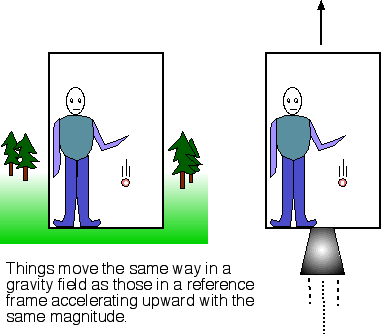Part of Einstein’s genius was his ability to look at ordinary things from a whole new perspective and logically follow through on the consequence of the insights he gained from his new perspective. He proposed an experiment involving two elevators: one at rest on the ground on the Earth and another, far out in space away from any planet, moon, or star, accelerating upward with an acceleration equal to that of one Earth gravity (9.8 meters/second2). (Modern readers can substitute “rocket ship” for Einstein’s elevator.) If a ball is dropped in the elevator at rest on the Earth, it will accelerate toward the floor with an acceleration of 9.8 meters/second2. A ball released in the upward accelerating elevator far out in space will also accelerate toward the floor at 9.8 meters/second2. The two elevator experiments get the same result!

Einstein used this to formulate the equivalence principle that would be the foundation of General Relativity. It states that “there is no experiment a person could conduct in a small volume of space that would distinguish between a gravitational field and an equivalent uniform acceleration.” A consequence of this is that if an elevator is falling freely toward the ground because of gravity, an occupant inside will feel weightless just as if the elevator was far away from any planet, moon, or star. No experiment would help you distinguish between being weightless far out in space and being in free-fall in a gravitational field.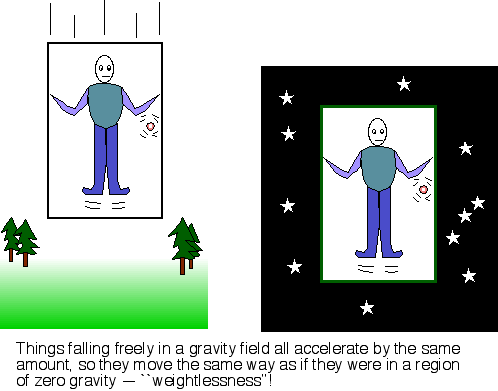Now suppose someone “at rest” outside your elevator way out in space shines a flashlight horizontally across the elevator you occupy toward the far wall of the elevator. If your elevator is at rest, then you will see the beam of light travel in a straight horizontal line. If your elevator is moving at a constant velocity upward relative to the person outside, you will see the beam of light travel in a straight-line path angled downward. The person outside still sees the beam traveling in a horizontal direction. If the elevator is accelerating upward, then the beam will follow a curved path downward relative to you. But if the beam of light curves in the accelerating elevator, then the equivalence principle says that the beam of light should also follow a curved path in a gravitational field.Light travels along the shortest path between two points in spacetime (a geodesic). If the geodesic is curved, then the path of light is curved. Einstein proposed in his General Relativity theory that what is called gravity is really the result of curved spacetime.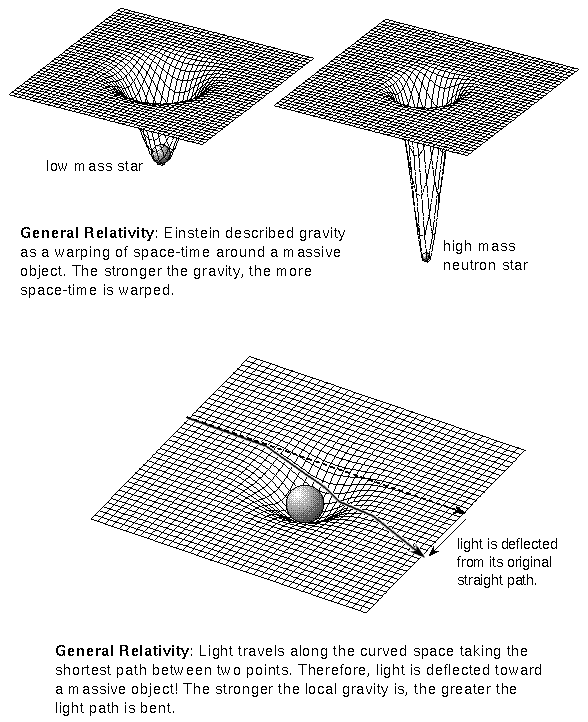The Earth does not orbit the Sun because the Sun is pulling on it. The Earth is simply following the shortest path in four-dimensional spacetime.

If you have ever taken a long flight, you probably already know that the shortest distance between two cities is not a straight line. Non-stop flights from the United States to Europe fly over parts of Greenland. On a flat map the plane’s flight path looks curved, but on a globe, that path is the shortest one! Light travels along a geodesic path between two points in spacetime. Far from any gravity source, the shortest distance is a straight line in three-dimensional space. Near a massive object, the shortest distance is curved in three-dimensional space. Stephen Hawking gives the nice analogy that what we see is like the curved motion of a shadow on the ground from a plane flying in a straight line over hilly terrain.

Einstein’s theory of General Relativity is a continuation or extension of Newton’s law of gravity. Einstein’s theory is not perfect (no scientific theory is absolutely perfect), but it does give a better understanding of the universe. In weak gravity conditions, they will give essentially the same results or predictions. Newton’s law of gravity assumes that the geometry of spacetime is flat while Einstein’s General Relativity allows any geometry to apply to spacetime. In weak gravity conditions, the curvature of spacetime is so small that Newton’s law of gravity works just fine. Since the mathematics of Newton’s laws of motion and gravity are simpler than for Einstein’s relativity theories, scientists prefer to use Newton’s law of gravity for understanding interactions of slow-moving objects in any weak gravity field. As mentioned at the beginning of the chapter, scientists use Newton’s laws of motion and gravity to very accurately guide spacecraft in our solar system. For very strong gravitational fields, Newton’s description of gravity becomes inadequate. Einstein’s theory of General Relativity must be used to describe the gravitational effects.

## 6.3 Evidence of Warped Spacetime

If Einstein’s theory of General Relativity is an accurate description of gravity, then there are some bizarre consequences. In this section the implications of General Relativity’s claim that gravity is the warping of spacetime will be explored in a prediction-observation format. A scientific theory must make testable predictions which are tested through observations and experiments.

1. Prediction: Light passing close to a massive object should be noticeably bent. The amount of bending increases as the mass increases.

Observation: During a solar eclipse you see that the stars along the same line of sight as the Sun are shifted “outward.” This is because the light from the star behind the Sun is bent toward the Sun and toward the Earth. The light comes from a direction that is different from where the star really is. But wouldn’t Newton’s law of gravity and the result from Einstein’s Special Relativity theory that E = mc2 predict light deflection too? Yes, but only half as much. General Relativity says that time is also stretched so the deflection is twice as great.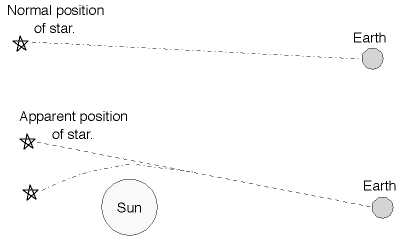Observation: The light from quasars is observed to be bent by gravitational lenses produced by galaxies between the Earth and the quasars. It is possible to see two or more identical images of the same background quasar. In some cases the light from background quasars or galaxies can be warped to form rings. Since the amount of warping depends on the mass of the foreground galaxy, you can estimate the total mass of the foreground galaxy.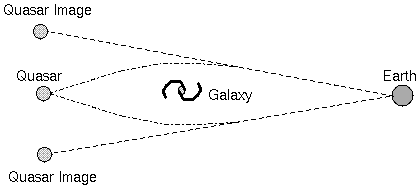The Einstein Cross (Q2237+0305) is formed by a foreground galaxy lensing the light from a background quasar into 4 images.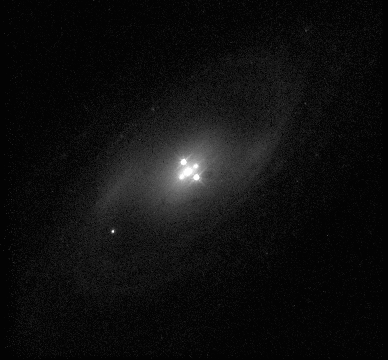Image courtesy of Bill Keel. Galaxy nucleus produces the image. Zoomed-in image of nucleus on right.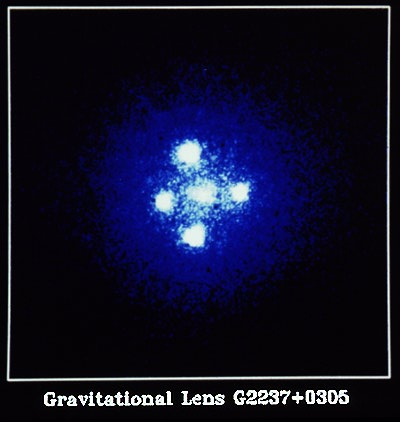Image courtesy of the Space Telescope Science Institute.

Below is a picture from the Hubble Space Telescope showing the lensing of a background galaxy by a cluster of galaxies in front. The distorted blue arcs visible around the center of the picture are the lensed background galaxy. If you select the image, an enlarged version will appear (courtesy of the Space Telescope Science Institute).2. Prediction: Time should run “slower” near a large mass. This effect is called time dilation.For example, if someone on a massive object (call her person A) sends a light signal to someone far away from any gravity source (call him person B) every second according to her clock on the massive object, person B will receive the signals in time intervals further apart than one second. According to person B, the clock on the massive object is running slow.

Observation: a) Clocks on planes high above the ground run faster than those on the ground. The effect is small since the Earth’s mass is small, so atomic clocks must be used to detect the difference. b) The Global Positioning Satellite (GPS) system must compensate for General Relativity effects or the positions it gives for locations would be significantly off.

3. Prediction: Light escaping from a large mass should lose energy — the wavelength must increase since the speed of light is constant. Stronger surface gravity produces a greater increase in the wavelength.This is a consequence of time dilation. Suppose person A on the massive object decides to send light of a specific frequency f to person B all of the time. So every second, f wave crests leave person A. The same wave crests are received by person B in an interval of time interval of (1+z) seconds. He receives the waves at a frequency of f/(1+z). Remember that the speed of light c = (the frequency f) × (the wavelength l). If the frequency is reduced by (1+z) times, the wavelength must INcrease by (1+z) times: lat B = (1+z) × lat A. In the Doppler effect, this lengthening of the wavelength is called a redshift. For gravity, the effect is called a gravitational redshift.

Observation: Spectral lines from the top layer of white dwarfs are significantly shifted by an amount predicted for compact solar-mass objects. The white dwarf must be in a binary system with a main sequence companion so that the amount the total shift due to the ordinary Doppler effect can be determined and subtracted out. Inside a black hole’s event horizon, light is redshifted to an infinitely long wavelength.4. Prediction: objects with mass should create ripples in the surrounding spacetime as they move, called gravitational waves.These waves do not travel through spacetime, but are the oscillations of spacetime itself! The spacetime ripples move at the speed of light. However, the waves are very small and extremely hard to detect.

Observation: even the most sensitive detectors have not yet directly detected the tiny stretching-shrinking of spacetime caused by a massive object moving. However, the decaying orbits of a binary pulsar system discovered in 1974 by Russell Hulse and Joseph Taylor can only be explained by gravity waves carrying away energy from the pulsars as they orbit each other. This observation provides a very strong gravity field test of General Relativity.The two pulsars in the binary system called PSR1913+16 orbit each other very rapidly with a period of only 7.75 hours in very eccentric and small elliptical orbits that bring them as close as 766,000 kilometers and then move them rapidly to over 3.3 million kilometers apart. Because of their large masses (each greater than the Sun’s mass) and rapidly changing small distances, the gravity ripples should be noticeable. Hulse and Taylor discovered that the orbit speed and separation of PSR1913+16 changes exactly in the way predicted by General Relativity. They were awarded the Nobel Prize in physics for this discovery.

The two pulsars in the binary system called PSR1913+16 orbit each other very rapidly with a period of only 7.75 hours in very eccentric and small elliptical orbits that bring them as close as 766,000 kilometers and then move them rapidly to over 3.3 million kilometers apart. Because of their large masses (each greater than the Sun’s mass) and rapidly changing small distances, the gravity ripples should be noticeable. Hulse and Taylor discovered that the orbit speed and separation of PSR1913+16 changes exactly in the way predicted by General Relativity. They were awarded the Nobel Prize in physics for this discovery.

The Laser Interferometer Gravitational-Wave Observatory (LIGO) is an international effort to directly detect the very small ripples in spacetime passing through the Earth. The basic LIGO set up involves two several-kilometer long arms perpendicular to each other. At either end of the arms are mirrors that bounce laser light back and forth many times and then are recombined to make an interference pattern of light and dark fringes as different parts of a wave’s heights and troughs cancel out or combine with another wave’s heights and troughs to varying degrees. Without any spacetime ripples, the laser light waves from the two arms will perfectly cancel each other out, so nothing will reach a photodetector. A gravity wave passing through a LIGO site, will stretch one of the arms while squeezing the other arm and then reverse the effect as the gravity waves passes by. This will make the combined light from the two arms get out of phase with each other, resulting in some of the laser light reaching the photodetector. The photodetector then measures how the light fluctuates over time. The technological challenge is to detect minute changes in the length of the arms as small as 10-18 meters (many times smaller than a proton!) in the midst of all of the terrestrial “noise” such as seismic events, sound waves, random laser fluctuations, and thermal effects of the materials used that can easily overwhelm the very weak gravity wave ripples of spacetime. A schematic for the United States version is shown below (adapted from a diagram in a slideset from Rainer Weiss).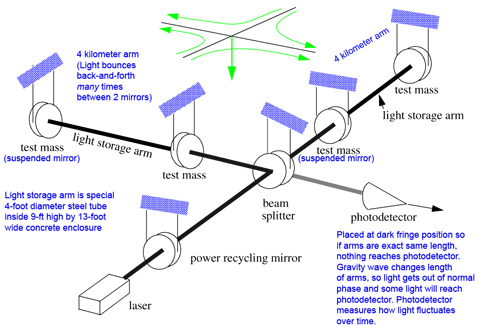LIGO sites are located in the United States (at Hanford, WA and Livingston, LA), in Germany (near Hannover), in Italy (near Pisa), in Japan (at the Kamioka mine), and a future site in Australia. The initial run of operations completed in 2007 without any detection of the waves. An additional run using enhanced detectors was completed in 2010 also without any detection of the waves. These negative results were not surprising, however, as the technology innovations needed in hardware and software are still being developed along with our ability to analyze the weak waves amidst all of the noise. Advanced LIGO is now being installed at the two United States sites that will increase the sensitivity of gravity wave detection by a factor of ten times. Being able to detect gravity waves from sources ten times farther away means an increase of a thousand times the volume of space or a thousand times the number of possible sources. It is expected to begin operations in July 2013.

Physicists tested some other predictions of the General Relativity Theory with the Gravity Probe B spacecraft mission. The spacecraft was in a polar orbit (pole to pole) 400 miles above the Earth’s surface. Four gyroscopes with extremely precise, perfect quartz spheres kept the spacecraft aligned with a particular guide star. Because the Earth warps spacetime around it and the Earth should also “drag” the spacetime around it as it rotates, there should be a twisting of the local spacetime. This twisting and stretching of the local spacetime should cause the gyroscopes in Gravity Probe B to get out of alignment as it orbited the Earth. The twisting and stretching of spacetime by the Earth should be very small because of the Earth’s tiny mass, so the drifting of the gyroscopes on Gravity Probe B were very small and the instruments on the spacecraft had to be exquisitely precise to detect the slight twisting and stretching of the spacetime near the Earth. The spacecraft finished data collection in October 2005 and intensive analysis of the data began. In April 2007, the scientists announced that they detected the predicted shift caused by the stretching of spacetime (the “geodetic effect”). After another two and a half years of data analysis, they announced in September 2009 that they were able to extract out the much smaller effect caused by the twisting of spacetime (the “frame-dragging effect”).

## Chapter Review

### Vocabulary

• equivalence principle
• General Relativity
• gravitational lens
• gravitational redshift
• spacetime
• Special Relativity

### Review Questions

1. When are the unusual effects predicted by the Special Relativity theory particularly noticeable? Has this theory been tested? If yes, how so? If not, why not?
2. What two things change when objects move at high speeds?
3. How does the speed of light depend on the observer’s location and motion?
4. Why is the speed of light the fastest that anything can travel?
5. How does the equivalence principle lead to the conclusion that spacetime is warped?
6. What did Einstein predict would happen to a light ray passing close to a massive object?
7. When are the unusual effects predicted by the General Relativity theory particularly noticeable? Have astronomers been able to test this theory of Einstein? If yes, how so? If not, why not?
8. How is Einstein’s theory of gravity the same as Newton’s law of gravity?